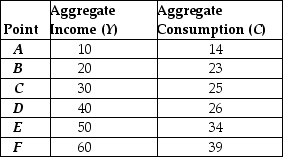# [Solved] Refer to the Information Provided in Table 21

Question 87
Multiple Choice

## Refer to the information provided in Table 21.2 below to answer the questions that follow. Table 21.2The data in the table was used to estimate the following consumption function: C = 12 + 0.4Y. -Refer to Table 21.2. The SUM that is calculated from all the error points from the table is equal to

A) -5.
B) +13.
C) +25.
D) +31.

10+ million students use Quizplus to study and prepare for their homework, quizzes and exams through 20m+ questions in 300k quizzes.

Explore our library and get Economics Homework Help with various study sets and a huge amount of quizzes and questions

3.5K

Study sets

66.5K

Quizzes

5.9M

Questions

Upload material to get free accessInvite a friend and get free accessSubscribe and get an instant access